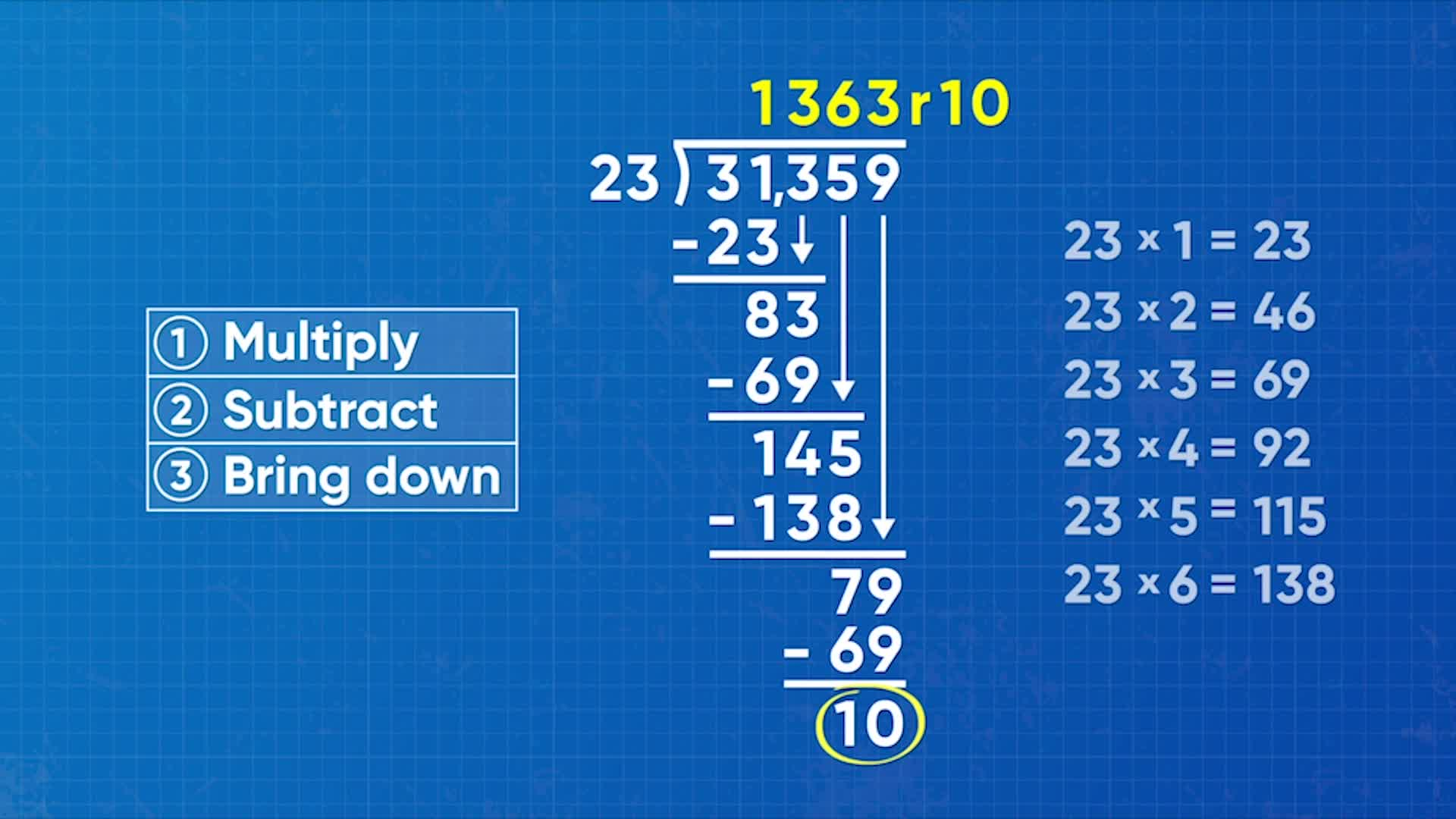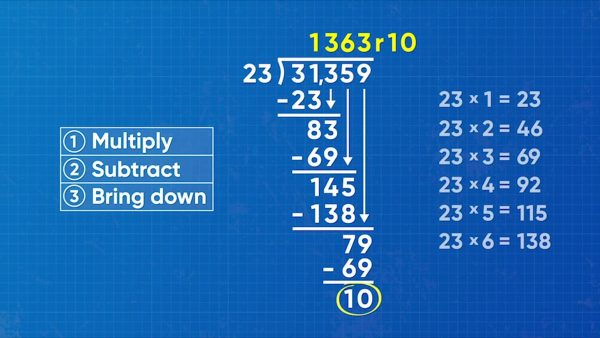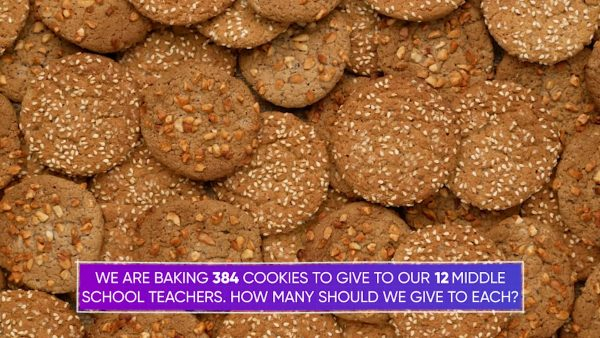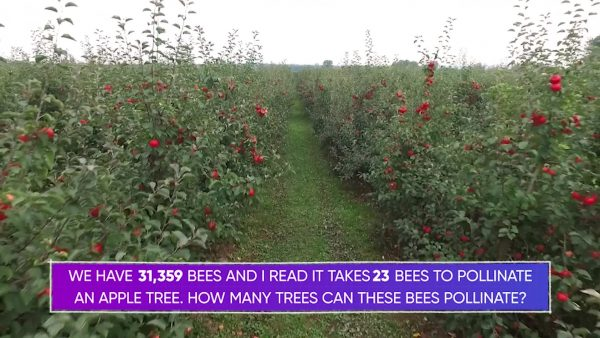Long Division (Standard Algorithm for Division) Math Video - 6th-8th Grade
1%
It was processed successfully!WHAT IS THE STANDARD ALGORITHM FOR LONG DIVISION?

The standard algorithm for long division gives a quick and efficient process to divide any two numbers. The process is the same no matter what numbers you use.

To better understand long division…

WHAT IS THE STANDARD ALGORITHM FOR LONG DIVISION?. The standard algorithm for long division gives a quick and efficient process to divide any two numbers. The process is the same no matter what numbers you use. To better understand long division…

## LET’S BREAK IT DOWN!

### Divide a 3-Digit Number by a 1-Digit NumberA batch of elephant toothpaste needs 936 milligrams of hydrogen peroxide divided equally among 4 containers. Divide 936 ÷ 4 to find how many milligrams go into each container. The standard algorithm for long division is a series of steps repeated in this order: divide, multiply, subtract, bring down. With the standard algorithm, we solve division problems one place value at a time. Start with the 9 in 938. Multiply: What multiple of 4 is closest to (but less than) 9? 4 × 2 = 8. Record a 2 in the hundreds place above the 9. Subtract: 9 -8 =1. Bring down: the next digit in 938 is 3. Write it next to the 1 to make 13. Repeat all the steps. 4 × 3 = 12, which is closest to 13. Write a 3 in the tens place above the line. Subtract 13 – 12 to get 1. Bring down the last digit, 6 to make 16. Repeat the steps again. 4 × 4 = 16. Write a 4 above the ones place. 16 – 16 = 0. There are no digits left to bring down, so you are done! The number above the line is 234 and the last subtraction resulted in 0. 936 ÷ 4 = 234. Try this one yourself: What is 875 ÷ 7?

Divide a 3-Digit Number by a 1-Digit Number A batch of elephant toothpaste needs 936 milligrams of hydrogen peroxide divided equally among 4 containers. Divide 936 ÷ 4 to find how many milligrams go into each container. The standard algorithm for long division is a series of steps repeated in this order: divide, multiply, subtract, bring down. With the standard algorithm, we solve division problems one place value at a time. Start with the 9 in 938. Multiply: What multiple of 4 is closest to (but less than) 9? 4 × 2 = 8. Record a 2 in the hundreds place above the 9. Subtract: 9 -8 =1. Bring down: the next digit in 938 is 3. Write it next to the 1 to make 13. Repeat all the steps. 4 × 3 = 12, which is closest to 13. Write a 3 in the tens place above the line. Subtract 13 – 12 to get 1. Bring down the last digit, 6 to make 16. Repeat the steps again. 4 × 4 = 16. Write a 4 above the ones place. 16 – 16 = 0. There are no digits left to bring down, so you are done! The number above the line is 234 and the last subtraction resulted in 0. 936 ÷ 4 = 234. Try this one yourself: What is 875 ÷ 7?

### Divide a 4-Digit Number by a 1-Digit NumberYou want to complete a trip of 1,421 miles in 6 days. Divide 1,421 ÷ 6 to find how many miles you must drive each day. To divide using the standard algorithm, write 1,421 under the division symbol and 6 to the left. Use the steps multiply, subtract, and bring down to find the quotient. What multiple of 6 is closest to 14? 6 × 2 = 12. Write a 2 in the hundreds position. Subtract: 14 – 12 = 2. Bring down the next digit, 2, to make 22. What multiple of 6 is closest to 22? 6 × 3 = 18. Write 3 in the tens position. Subtract: 22 – 18 = 4. Bring down the next digit, 1, to make 41. What multiple of 6 is closest to 41? 6 × 6 = 36. Write 6 above the ones position. Subtract: 41 – 36 = 5. There are no more digits to bring down. 5 is less than 6 so 5 cannot be evenly divided into 6 groups. 1,421 ÷ 6 = 236 with a remainder of 5. You need to drive a little more than 236 miles each day. Try this one yourself: Divide 2,576 ÷ 8.

Divide a 4-Digit Number by a 1-Digit Number You want to complete a trip of 1,421 miles in 6 days. Divide 1,421 ÷ 6 to find how many miles you must drive each day. To divide using the standard algorithm, write 1,421 under the division symbol and 6 to the left. Use the steps multiply, subtract, and bring down to find the quotient. What multiple of 6 is closest to 14? 6 × 2 = 12. Write a 2 in the hundreds position. Subtract: 14 – 12 = 2. Bring down the next digit, 2, to make 22. What multiple of 6 is closest to 22? 6 × 3 = 18. Write 3 in the tens position. Subtract: 22 – 18 = 4. Bring down the next digit, 1, to make 41. What multiple of 6 is closest to 41? 6 × 6 = 36. Write 6 above the ones position. Subtract: 41 – 36 = 5. There are no more digits to bring down. 5 is less than 6 so 5 cannot be evenly divided into 6 groups. 1,421 ÷ 6 = 236 with a remainder of 5. You need to drive a little more than 236 miles each day. Try this one yourself: Divide 2,576 ÷ 8.

### Divide a 3-Digit Number by a 2-Digit numberYou want to divide a batch of 384 cookies among 12 middle school teachers. Solve 384 ÷ 12 using the standard algorithm for long division. The same process works no matter how many digits are in the divisor or dividend. Start by asking what multiple of 12 is less than or equal to 38? 12 × 3 = 36, so write 3 in the tens place and subtract to find the remainder: 38 – 36 = 2. Bring down the 4 to make 24. What multiple of 12 is closest to 24? 12 × 2 = 24, so write 2 in the ones position and subtract to find the remainder 24 – 24 = 0.There are no more digits to bring down. 384 ÷ 12 = 32. You can give each teacher 32 cookies. Yum! Try this yourself: What is 224 ÷ 32?

Divide a 3-Digit Number by a 2-Digit number You want to divide a batch of 384 cookies among 12 middle school teachers. Solve 384 ÷ 12 using the standard algorithm for long division. The same process works no matter how many digits are in the divisor or dividend. Start by asking what multiple of 12 is less than or equal to 38? 12 × 3 = 36, so write 3 in the tens place and subtract to find the remainder: 38 – 36 = 2. Bring down the 4 to make 24. What multiple of 12 is closest to 24? 12 × 2 = 24, so write 2 in the ones position and subtract to find the remainder 24 – 24 = 0.There are no more digits to bring down. 384 ÷ 12 = 32. You can give each teacher 32 cookies. Yum! Try this yourself: What is 224 ÷ 32?

### Divide a Large Multi-Digit Number by a Multi-Digit NumberIt takes 23 bees to pollinate one apple tree. If we have a total of 31,359 bees, how many apple trees can they pollinate? Solve 31,359 ÷ 23 by using the standard algorithm for long division. Consider the first two digits of 31,359. What multiple of 23 is closest to 31? 23 × 1 = 23, so write a 1 in the thousands place and subtract to find the difference: 31 – 23 = 8. Bring down the 3 to make 83. 23 × 3 = 69, so write 3 in the hundreds place and subtract to find the difference: 83 – 69 = 14. Bring down the 5 to make 145. 23×6=138, so write 6 in the tens place and subtract 145-138 =7. Bring down the 9 to make 79. 23×3=69, so write 3 in the ones place and subtract 79-69=10. There are no more digits to bring down and 10 cannot be divided into 23 equal groups. 31,359 ÷ 23 = 1,363 with a remainder of 10. The bees can pollinate 1,363 apple trees. Try this yourself: What is 53,922 ÷ 43?

Divide a Large Multi-Digit Number by a Multi-Digit Number It takes 23 bees to pollinate one apple tree. If we have a total of 31,359 bees, how many apple trees can they pollinate? Solve 31,359 ÷ 23 by using the standard algorithm for long division. Consider the first two digits of 31,359. What multiple of 23 is closest to 31? 23 × 1 = 23, so write a 1 in the thousands place and subtract to find the difference: 31 – 23 = 8. Bring down the 3 to make 83. 23 × 3 = 69, so write 3 in the hundreds place and subtract to find the difference: 83 – 69 = 14. Bring down the 5 to make 145. 23×6=138, so write 6 in the tens place and subtract 145-138 =7. Bring down the 9 to make 79. 23×3=69, so write 3 in the ones place and subtract 79-69=10. There are no more digits to bring down and 10 cannot be divided into 23 equal groups. 31,359 ÷ 23 = 1,363 with a remainder of 10. The bees can pollinate 1,363 apple trees. Try this yourself: What is 53,922 ÷ 43?

## LONG DIVISION VOCABULARY

Area model
A rectangular diagram or model used for multiplication and division problems, in which the factors or the quotient and divisor define the length and width of the rectangle. Also called the box method.
Partial quotients
A strategy for division where we subtract multiples of the divisor from the dividend until we get to 0, or as close to 0 as possible.
Algorithm
A procedure in mathematics or a description of a set of steps that we can use to solve a mathematical computation.
Long division
A process of dividing a number that has at least three digits by one that is two digits or more, sometimes leaving a remainder.
Standard algorithm for division
A series of repeated steps for performing division problems. The steps in order are: divide, multiply, subtract, bring down.
Partitioning
Division into equal parts.
In division, the amount or number to be divided.
In division, a number that divides another number, either completely or with a remainder.

## LONG DIVISION DISCUSSION QUESTIONS

### What are the four repeated steps of the standard algorithm for long division?

Divide, Multiply, Subtract, Bring down

When I divide, I can look at multiples of the divisor to help me find the dividend.

### How do you know when you are done with a long division problem?

When there are no more digits to bring down and I have a remainder of 0, or when there are no more digits to bring down and the remainder is smaller than the divisor.

### Why use a standard algorithm to divide?

The standard algorithm is a very efficient way to divide.

### How can you use the standard algorithm to find 4,564 ÷ 7?

I can start by making a list of multiples of 7. 4 is less than 7, so consider multiples of 7 close to 45. Multiply: 7 × 6 = 42. Subtract: 45 – 42 = 3. Bring down the 6 to make 36. Find multiples of 7 close to 36. Multiply: 7 × 5 = 35. Subtract: 36 – 35 = 1. Bring down the 4 to make 14. Find multiples of 7 close to 14. Multiply: 7 × 2 = 14. Subtract: 14 – 14 = 0. No numbers remain to bring down, and the remainder is 0. 4,564 ÷ 7 = 652.
X

## Success

We’ve sent you an email with instructions how to reset your password.
Ok

## Choose Your Free Trial Period### 7 DaysContinue to Lessons### 90 DaysGet 90 days free by inviting other teachers to try it too.

Share with Teachers

## Get 90 Days Free

### By inviting 4 other teachers to try it too.

4 required

Skip, I will use a 7 days free trial

## Thank You!

Enjoy your free 90 days trial